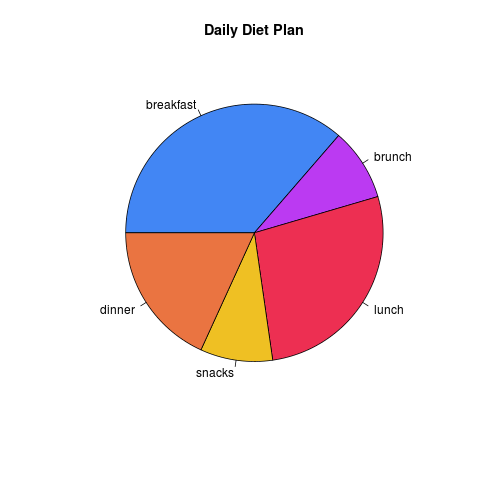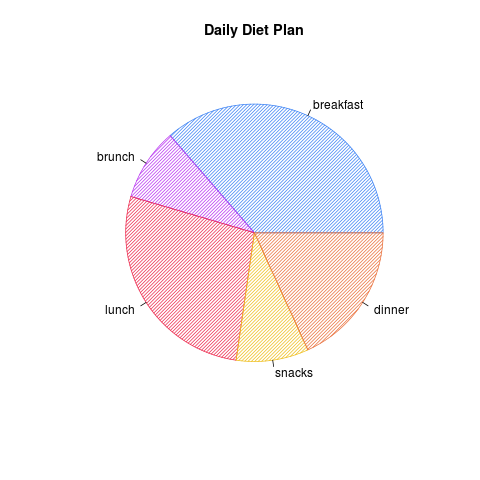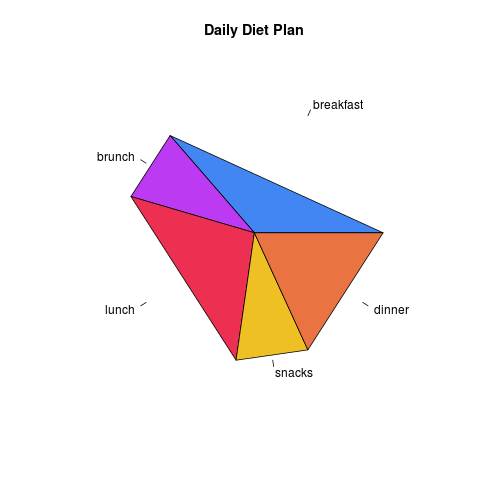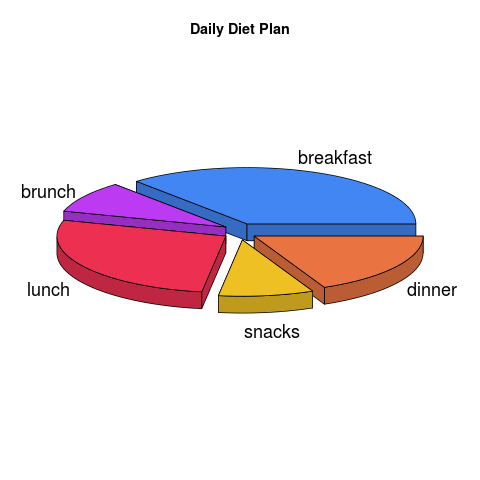Pie Chart is a pictorial representation of proportions in a whole as sectors in a circle.

## Draw Pie Chart in R programming language

R programming language provides two functions – pie() and pie3d() to draw pie charts.

### Syntax of R pie function

 pie(x, labels = NULL, edges = 200, radius = 0.8, clockwise = FALSE, init.angle = 0, density = NULL, angle = 0, col = NULL, border = NULL, lty = NULL, main = NULL, …)

where

• x [mandatory] is a numerical vector with values >=0. Each values decides the proportion of circle.
• labels is a character vector. They are names for the slices.
• edges the circular outline of the pie is approximated by a polygon with this many edges.
• radius of the circle in pie chart.
• clockwise represents the logical indicating if slices are drawn clockwise or counter clockwise. counter clockwise is default. {TRUE,FALSE}
• init.angle is the initial angle(in degrees) to start with for drawing sectors. [0,360]
• density of shading lines, in lines per inch
• angle is the slope of shading lines in degrees (counter-clockwise).
• col is a vector of colors to be used in filling or shading the slices.
• border, lty – arguments passed to polygon which draws each slice.
• main is an overall title for the plot.
• … is graphical parameters can be given as arguments to pie. They will affect the main title and labels only.

### Example 1 – Simple Pie Chart

When the above program is run, following pie chart is created at the location of your Rscript.### Example 2 – Pie Chart with Striped Lines### Example Pie Chart with Edged sectors### Syntax of R pie3D function

plotrix library is required to use it in an Rscript

x [mandatory] is a numerical vector with values >=0. Each values decides the proportion of circle.where

• labels is a character vector. They are names for the slices.
• radius of the circle in pie chart.
• col is a vector of colors to be used in filling or shading the slices.
• main is an overall title for the plot.
• explode is the amount by which sectors are exploded
• … extra arguments

### Example 1 – Simple 3D Pie Chart in R

When the above program is run, following pie chart is created at the location of your Rscript.### Conclusion :

In this R Tutorial, we have learnt to draw Pie Chart in R programming language in 2D and 3D.